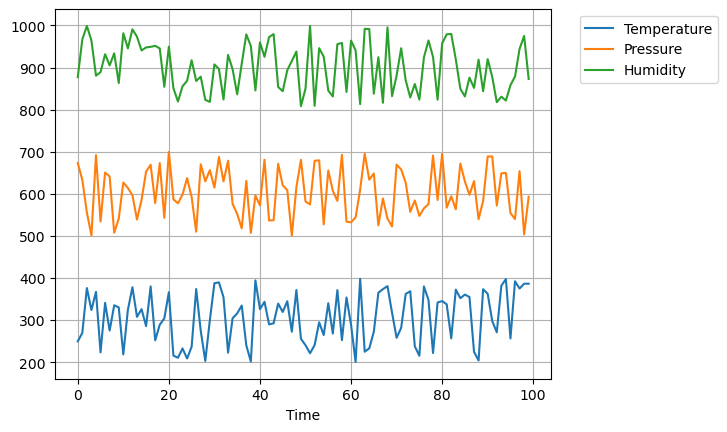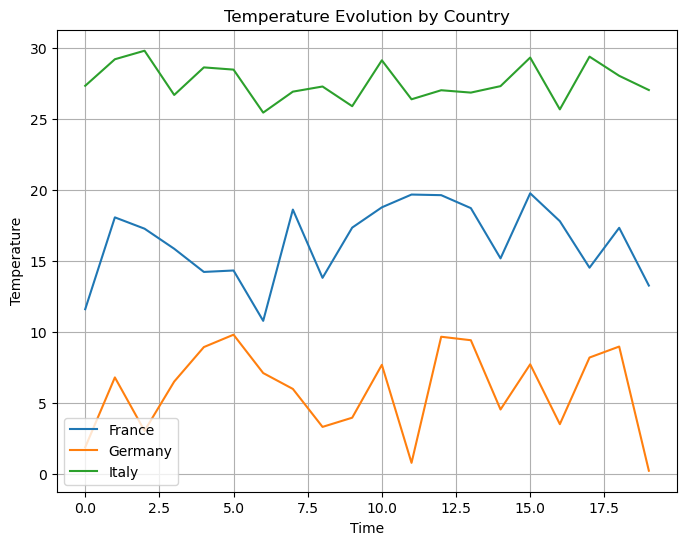## Libraries

Pandas is a popular open-source Python library used for data manipulation and analysis. It provides data structures and functions that make working with structured data, such as tabular data (like `Excel` spreadsheets or `SQL` tables), easy and intuitive.

To install Pandas, you can use the following command in your command-line interface (such as `Terminal` or `Command Prompt`):

`pip install pandas`

Matplotlib functionalities have been integrated into the pandas library, facilitating their use with `dataframes` and `series`. For this reason, you might also need to import the matplotlib library when building charts with Pandas.

This also means that they use the same functions, and if you already know Matplotlib, you'll have no trouble learning plots with Pandas.

``````import pandas as pd
import random, numpy as np # used for data generation
import matplotlib.pyplot as plt``````

## Line chart with multiple variables

### Dataset

In order to create graphics with Pandas, we need to use pandas objects: `Dataframes` and `Series`. A dataframe can be seen as an `Excel` table, and a series as a `column` in that table. This means that we must systematically convert our data into a format used by pandas.

Since line charts need quantitative variables, we will create a dataset with temperature, pressure and humidity, evolving through time.

``````# Define the number of time points
num_time_points = 100

# Generate time values
time_values = np.arange(num_time_points)

# Generate random data for three variables (e.g., temperature, pressure, and humidity)
temperature = np.random.uniform(200, 400, num_time_points)  # Random temperature values between 20 and 30
pressure = np.random.uniform(500, 700, num_time_points)  # Random pressure values between 900 and 1100
humidity = np.random.uniform(800, 1000, num_time_points)  # Random humidity values between 30 and 70

data = {
'Time': time_values,
'Temperature': temperature,
'Pressure': pressure,
'Humidity': humidity
}
df = pd.DataFrame(data)``````

### Create the plot

Once we've opened our dataset, we'll now create the graph. The following displays the evolution of our variables using the `plot()` function, and since we want the evolution of every variable in our pandas dataframe, we juste have to specify which variable will be in the `x-axis`, which is `'Time'`.

Also, keep in mind that the `kind='line'` argument is facultative (you can remove it!) since it's the default value when calling the `plot()` function.

``````# Define the size of the figure
plt.figsize=(8, 6)

# Create and display the linechart
df.plot(x='Time',
kind='line', # (facultative) Default argument
grid=True, # Add a grid in the background
)
plt.legend(loc='upper right',
bbox_to_anchor=(1.35, 1), # Shift the legend outside the chart (35% on the right)
)
plt.show()``````## Line chart with multiple groups

### Dataset

Now let's say we want to display the evolution of temperature only and for different rows. For this, we create a new dataset:

• random temperature data is generated using `np.random.uniform()`. This function creates random numbers within a specified range.
• `country_labels` is created using `np.repeat()`. It repeats the country names in the countries array so that each country name is associated with its corresponding temperature data points.
• `time_values` is generated using `np.tile()` and `np.arange()`. It creates a sequence of numbers from 0 to 19 (for the 20 data points per country) and then repeats this sequence for each country
``````# Define the number of data points
num_data_points_per_country = 20

# Generate random temperature data for each country
france_temperatures = np.random.uniform(10, 20, num_data_points_per_country)  # Temperature range for France (10-20 degrees)
germany_temperatures = np.random.uniform(0, 10, num_data_points_per_country)   # Temperature range for Germany (0-10 degrees)
italy_temperatures = np.random.uniform(25, 30, num_data_points_per_country)    # Temperature range for Italy (25-30 degrees)

# Create an array of country labels corresponding to the data points
countries = ['France', 'Germany', 'Italy']
country_labels = np.repeat(countries, num_data_points_per_country)

# Generate time values
time_values = np.tile(np.arange(num_data_points_per_country), len(countries))

# Create a Pandas DataFrame
data = {
'Country': country_labels,
'Temperature': np.concatenate([france_temperatures, germany_temperatures, italy_temperatures]),
'Time': time_values
}

df = pd.DataFrame(data)``````

### Create the plot

We begin by grouping the data by country, then creates a figure and axis for plotting. For each country, we plot the temperature against time as separate lines on the same graph, adds labels, a title, a legend for country identification, and a grid for clarity.

``````# Group the data by continent and calculate the average life expectancy for each year
df_country = df.groupby(['Country'])

# Create a figure and axis object using the object-oriented approach
fig, ax = plt.subplots(figsize=(8, 6))

# Plot each group as a separate line
for key, group in df_country:
ax.plot(group['Time'], group['Temperature'], label=key)

# Set axis labels and title
ax.set_xlabel('Time')
ax.set_ylabel('Temperature')
ax.set_title('Temperature Evolution by Country')

ax.legend()

# Display the grid
ax.grid(True)

# Show the plot
plt.show()``````## Going further

This post explains how to create a line chart with mutliple variables and groups, with pandas.

For more examples of how to create or customize your line charts, see the line charts section. You may also be interested in how to customize a line chart with pandas.

## Contact & Edit

👋 This document is a work by Yan Holtz. You can contribute on github, send me a feedback on twitter or subscribe to the newsletter to know when new examples are published! 🔥